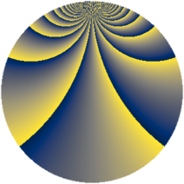# Properties

 Label 539.2.rLevel $539$ Weight $2$ Character orbit 539.r Rep. character $\chi_{539}(23,\cdot)$ Character field $\Q(\zeta_{21})$ Dimension $552$ Newform subspaces $2$ Sturm bound $112$ Trace bound $3$

# Related objects

## Defining parameters

 Level: $$N$$ $$=$$ $$539 = 7^{2} \cdot 11$$ Weight: $$k$$ $$=$$ $$2$$ Character orbit: $$[\chi]$$ $$=$$ 539.r (of order $$21$$ and degree $$12$$) Character conductor: $$\operatorname{cond}(\chi)$$ $$=$$ $$49$$ Character field: $$\Q(\zeta_{21})$$ Newform subspaces: $$2$$ Sturm bound: $$112$$ Trace bound: $$3$$ Distinguishing $$T_p$$: $$2$$

## Dimensions

The following table gives the dimensions of various subspaces of $$M_{2}(539, [\chi])$$.

Total New Old
Modular forms 696 552 144
Cusp forms 648 552 96
Eisenstein series 48 0 48

## Trace form

 $$552 q + 2 q^{3} + 44 q^{4} + 4 q^{5} - 20 q^{6} - 2 q^{7} + 12 q^{8} + 12 q^{9} + O(q^{10})$$ $$552 q + 2 q^{3} + 44 q^{4} + 4 q^{5} - 20 q^{6} - 2 q^{7} + 12 q^{8} + 12 q^{9} - 6 q^{10} - 76 q^{12} + 16 q^{13} - 70 q^{14} + 12 q^{15} + 36 q^{16} - 6 q^{17} - 10 q^{18} - 2 q^{19} - 40 q^{20} + 2 q^{21} + 8 q^{24} + 42 q^{25} + 10 q^{26} - 16 q^{27} - 16 q^{28} - 52 q^{29} - 32 q^{30} - 78 q^{31} - 116 q^{32} + 4 q^{33} - 108 q^{34} + 6 q^{35} - 112 q^{36} - 46 q^{37} + 8 q^{38} + 4 q^{39} - 112 q^{40} + 48 q^{41} - 8 q^{42} + 20 q^{43} - 32 q^{44} - 6 q^{45} + 126 q^{46} - 62 q^{47} + 128 q^{48} + 24 q^{49} - 188 q^{50} - 32 q^{51} + 114 q^{52} + 26 q^{53} + 142 q^{54} - 16 q^{55} - 76 q^{56} - 18 q^{57} + 22 q^{58} + 64 q^{59} - 44 q^{60} - 54 q^{61} - 20 q^{62} - 116 q^{63} - 92 q^{64} - 14 q^{65} - 72 q^{66} - 24 q^{67} - 16 q^{68} - 36 q^{69} - 158 q^{70} - 62 q^{71} - 260 q^{72} - 14 q^{73} - 12 q^{74} - 218 q^{75} - 114 q^{76} + 2 q^{77} - 54 q^{78} - 12 q^{79} - 172 q^{80} - 198 q^{81} + 38 q^{82} + 66 q^{83} - 180 q^{84} - 48 q^{85} + 130 q^{86} + 6 q^{87} - 14 q^{89} + 500 q^{90} + 118 q^{91} + 22 q^{92} + 202 q^{93} + 242 q^{94} - 44 q^{95} + 254 q^{96} + 4 q^{97} - 12 q^{98} + O(q^{100})$$

## Decomposition of $$S_{2}^{\mathrm{new}}(539, [\chi])$$ into newform subspaces

Label Dim $A$ Field CM Traces $q$-expansion
$a_{2}$ $a_{3}$ $a_{5}$ $a_{7}$
539.2.r.a $276$ $4.304$ None $$0$$ $$-1$$ $$-2$$ $$-2$$
539.2.r.b $276$ $4.304$ None $$0$$ $$3$$ $$6$$ $$0$$

## Decomposition of $$S_{2}^{\mathrm{old}}(539, [\chi])$$ into lower level spaces

$$S_{2}^{\mathrm{old}}(539, [\chi]) \cong$$ $$S_{2}^{\mathrm{new}}(49, [\chi])$$$$^{\oplus 2}$$